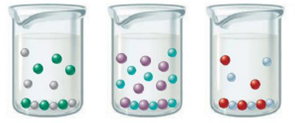Chapter 16, Problem 8Q

Chapter
Section
Textbook Problem

# For which of the following is the Ksp value of the ionic compound the largest? The smallest? Explain your answer.Interpretation Introduction

Interpretation: The ionic compound with largest Ksp value and the compound with smallest Ksp value is to be identified from the given figures. An explanation is to be given for the choice made.

Concept introduction: The solubility product, Ksp is an equilibrium product that is applied when salt partially dissolve in a solvent. If the number of ions is same, then value of Ksp is directly compared to calculate its solubility. Higher the concentration of ions, larger will be the Ksp value.

Explanation

Explanation

To determine: The ionic compound with largest Ksp value and the compound with smallest Ksp value; the reason of the choice made.

The diagram of given compounds is,

Figure 1

The number of cations and anions in figure (I),

Cations-3Anions-3

The number of cations and anions in figure (II),

Cations-5Anions-5

The number of cations and anions in figure (III),

Cations-2Anions-2

Solubility product, Ksp is defined as the concentration of ions in a saturated solution where each ion is raised to the power of their coefficients. An increase in solubility leads to increase in the formation of ions.

For example,

MX(s)M+(aq)+X(aq)

Where,

• MX is a binary compound

### Still sussing out bartleby?

Check out a sample textbook solution.

See a sample solution

#### The Solution to Your Study Problems

Bartleby provides explanations to thousands of textbook problems written by our experts, many with advanced degrees!

Get Started